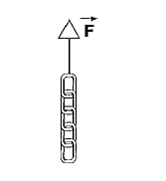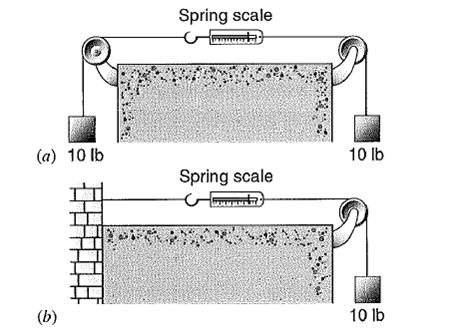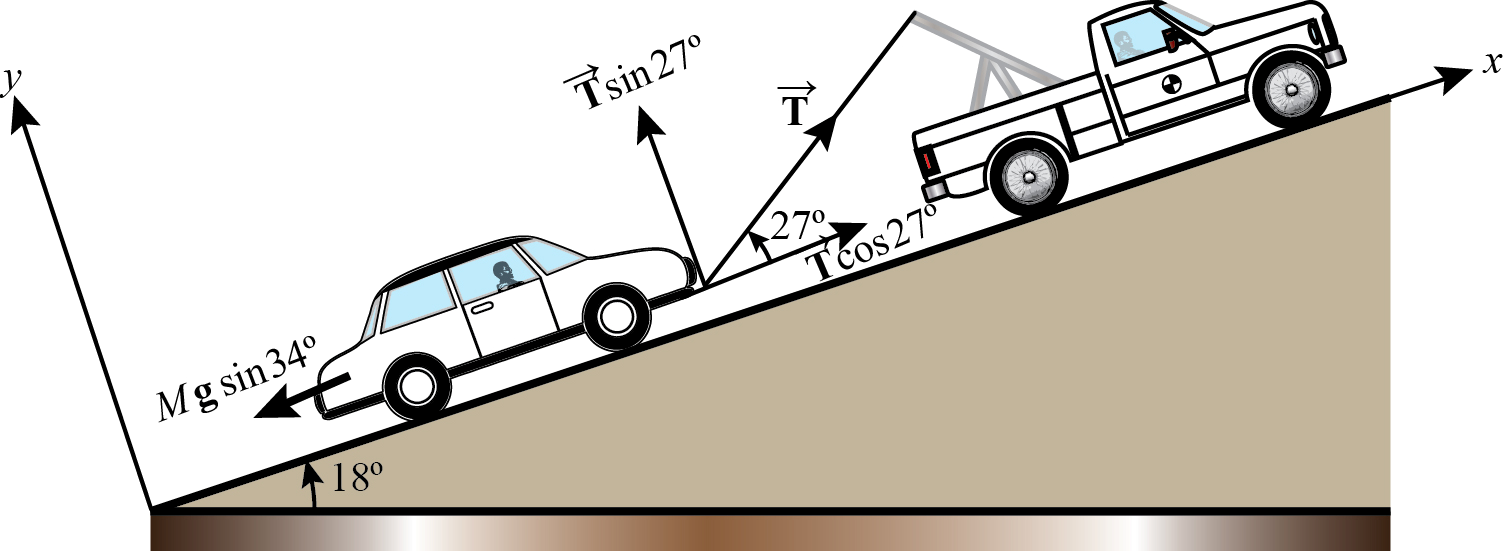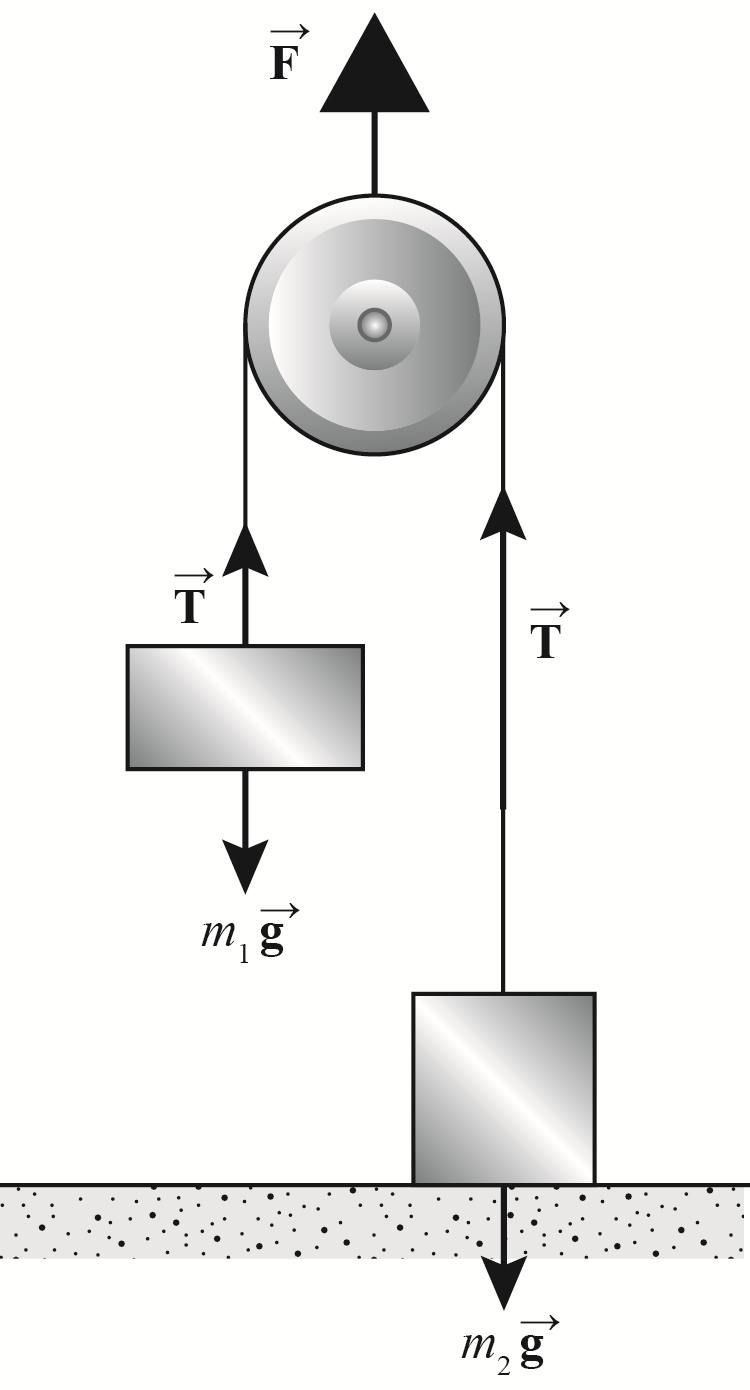×#### Thank you for registering.

One of our academic counsellors will contact you within 1 working day.

Click to Chat

1800-1023-196

+91-120-4616500

CART 0

• 0

MY CART (5)

Use Coupon: CART20 and get 20% off on all online Study Material

ITEM
DETAILS
MRP
DISCOUNT
FINAL PRICE
Total Price: Rs.

There are no items in this cart.
Continue Shopping• Complete JEE Main/Advanced Course and Test Series
• OFFERED PRICE: Rs. 15,900
• View Details

```Solved Examples on Laws of Motion

Question 1: A chain consisting of five links, each with mass 100 g, is lifted vertically with a constant acceleration of 2.50 m/s2, as shown in below figure. Find,

(b) The force F exerted on the top link by the agent lifting the chain.

(c) The net force on each link.Concept:

Force acting on the object (F) is equal to the product of mas of the (m) and acceleration of the auto mobile (a).

So, Fnet = ma

Weight of the automobile (W) is equal to the product of mass of the automobile (m) and acceleration due to gravity on the surface of the earth (g = 9.81 m/s2).

W = mg

Solution:

(a) The net force on each link is the same. To obtain the net force Fnet on each link, substitute 0.100 kg for m and 2.50 m/s2 for a in the equation Fnet = ma,

Fnet = ma

=(0.100 kg)(2.50 m/s2)

=(0.250 kg.m/s2) (1 N/1 kg.m/s2)

=0.250 N

To obtain the weight W of the each link, substitute 0.100 kg for m and 9.81 m/s2 for g in the equation W = mg,

W = mg

=(0.100 kg)( 9.81 m/s2)

= (0.981 kg.m/s2) (1 N/1 kg.m/s2)

=0.981 N

On each link (except the top or bottom link) there is a weight W, an upward force U from the link above, and a downward force D from the link below.

So, Fnet = U-D-W

Then, U= Fnet+W+D

=(0.250 N)+(0.981 N)+D

=1.23 N+D

For the bottom link, D=0 .

So, U=1.23 N+D

=1.23 N+0

=1.23 N

U = 1.23 N+1.23 N

= 2.46 N

U = 1.23 N+2.46 N

= 3.69 N

U = 1.23 N+3.69 N

= 4.92 N

From the above observation we conclude that, the force F exerted on the top link by the agent lifting the chain would be 4.92 N.

(b) To obtain the upward force U for the top link, substitute 4.92 N for D in the equation U=1.23 N+D,

U=1.23 N+D

=1.23 N+4.92 N

=6.15 N

From the above observation we conclude that, the upward force U for the top link would be 6.15 N.

(c) The net force on each link is the same. To obtain the net forces Fnet acting between adjacent on each link, substitute 0.100 kg for m and 2.50 m/s2 for a in the equation Fnet = ma,

Fnet = ma

=(0.100 kg)(2.50 m/s2)

=(0.250 kg.m/s2) (1 N/1 kg.m/s2)

=0.250 N

From the above observation we conclude that, the forces acting between adjacent links would be 0.250 N.Question 2:  (a) Two 10-lb weights are attached to a spring scale as shown in Fig. 1a. What is the reading of the scale?

(b) A single 10-lb weight is attached to a spring scale which itself is attached to a wall, as shown in figure 1b. What is the reading of the scale? (Ignore the weight of the scale.)Solution:

a) Let W1 is the weight of the left side block (W1 = 10-lb), a1 is the upward acceleration of the block and m1 is the mass of the block of the left hand side.

The upward force due to the upward acceleration a1 will be,

F1= m1a1       …… (1)

If T is the tension in the upward direction on the left side block (shown in the free body diagram), then the net force will be,

m1a1= W1-T     …… (2)

Let W2 is the weight of the right side block (W2 = 10-lb), a2 is the downward acceleration of the block and m2 is the mass of the block of the right hand side.

The downward force due to the downward acceleration a2 on the right side block will be,

F2= m2a2       …… (3)

If T is the tension in the upward direction on the left side block (shown in the free body diagram), then the net force will be,

m2a2= W2-T     …… (4)

FBD:-

Subtracting equation (4) from equation (2),

m1a1- m2a2 = W1-T –T+ W2     …… (5)

Since, W1= W2=10-lb and there is no relative motion, the left side of the equation (5) will be zero.

So, W1-T –T+ W2= 0

2T = (W1 + W2)

= (10-lb+10-lb)

So, T = 20-lb/2

=10-lb         …… (6)

From equation (6) we observed that, the reading of the scale will be 10-lb.

b) When a single 10-lb weight (W=10-lb) is attached to a spring scale which itself is attached to a wall, the net exerted force (Fnet) on the block will be equal to weight of the block (W) minus tension exerted on the block (T).

So, Fnet = W-T        …… (7)

Since the weight is attached to a spring scale which itself is attached to a wall, therefore the net force will be zero (Fnet=0).

Putting the value of Fnet in equation (7), we get,

W-T=0

T = W

= 10-lb      …… (8)

From equation (8) we observed that, the reading of the scale will be 10-lb.

Question 3:-  A 1200-kg car is being towed up an 18° incline by means of a rope attached to the rear of a truck. The rope makes an angle of 27° with the incline. What is the greatest distance that the car can be towed in the first 7.5 s starting from rest if the rope has a breaking strength of 4.6 kN? Ignore all the resistive forces on the car. See the low figure.Concept:

The figure below shows the car of mass M towed by another car through a rope of breaking strength T.Assume that the x axis is along the length of the ramp and y axis is perpendicular to it such that the tension in the rope along the x axis is whereas the tension in the rope along the y axis is .

Apply Newton’s second law of motion along the x axis,

T cos (27°) – Mg sin (18°) = Max

Here ax is the acceleration of the car of mass M along the x axis and g is the acceleration due to gravity.

ax = T cos (27°) – Mg sin (18°)/M

From this acceleration, one can calculate the distance travelled by the car in time t using relation,

x = ½ axt2

Solution:

Substitute 1200 kg for M, 9.8 m/s2  for g and 4.6 kN for T in equation,

ax = [T cos (27°) – Mg sin (18°)]/M

ax = [(4.6 kN) (103 N/1 kN) cos (27°) – (1200 kg) (9.81 m/s2)sin (18°)]/[1200 kg]

= {(4098.6 N) [(1 kg.m/s2)/1 N]/[1200 kg]}-3.02 m/s2

= 3.41 m/s2 - 3.02 m/s2

= 0.38 m/s2

Therefore, the acceleration of the car towed up by another car with a rope of breaking strength 4.6 kN is 0.38 m/s2.

Substitute 0.38 m/s2 for ax and 7.5 s for t in equation x = ½ axt2,

x = ½ (0.38 m/s2) (7.5 s)2

= 10.8 m

Therefore, the distance by which the car can move in 7.5 s is 10.8 m.

Question 4:- Someone exerts a force F directly up on the axle of the pulley shown in below figure. Consider the pulley and string to be mass less and the bearing frictionless. Two objects, m1 of mass 1.2 kg and m2 of mass 1.9 kg, are attached as shown to the opposite ends of the string, which passes over the pulley. The object m2 is in contact with the floor. (a) What is the largest value of force may have so that m2 will remain at rest on the floor? (b) What is the tension in the string if the upward force F is 110 N? (c) With the tension determined in part (b), what is the acceleration of m1?Concept:

The figure below shows the forces acting in the block-pulley system:It is important to note that the tension pulling the pulley down is 2T whereas the force pushing it upwards is F. Therefore, the block with mass m2 will stay on floor when

F ≤ 2T, and the tension in the string T is equal to the weight of the block on floor.

The net force on block with mass m1 is given as:

Fnet = F-m1g

Thus, the acceleration of block with mass m1 is:

a = (T-m1g)/m1

Solution:

(a) Substitute 1.9 kg for m2, and 9.8 m/s2 for g in equation T = m2g,

T = (1.9 kg) (9.8 m/s2)

= (18.62 kg. m/s2) [1 N/(1kg. m/s2)]

= 18.62 N

The maximum force F for which the block stays on the floor is F= 2T.

Substitute 18.62 N for T in equation F = 2T,

F = 2 (18.62 N)

= 37.24 N

Round off to two significant figures

F = 37 N

Therefore, the maximum force F for which block with mass m2 stays on floor is 37 N.

(b) The tension in the string is related to upward force as:

T = F/2

Substitute 110 N for F,

T = F/2

= 110 N/2

= 55 N

Therefore, the tension in the string corresponding to upward force of 110 N is 55 N.

(c) Substitute 55 N for T, 1.2 kg  for m1 and 9.8 m/s2 for g in equation a = T-m1g/m1

a = [55 N- (1.2 kg) (9.8 m/s2)]/1.2 kg

= {(55 N) [(1 kg.m/s2)/1 N]- (11.76 kg.m/s2)}/1.2 kg

= 36 m/s2

Therefore, the acceleration of the block with mass m1 is 36 m/s2.

Question 5:- The coefficient of static friction between the tires of a car and a dry road is 0.62. The mass of the car is 1500 kg. What maximum braking force is obtainable

(a) on a level road and

Concept:

Assume that the mass of the car is m and the coefficient of friction between the tires of the car and the road is µs.

The magnitude of maximum braking force obtainable on the level road is equal to the magnitude of the static frictional force between the tires and the road. The magnitude of the frictional force is,

f = µs N

Here N is the magnitude of normal force on the car.

(a) The car is in vertical equilibrium on the level road therefore the normal force on the car should be equal to its weight (mg) in magnitude, and must act in opposite direction, that is

N = mg

Substitute N = mg  in equation f = µs N ,

f = µs N

= µsmg

Therefore, the magnitude of maximum obtainable braking force is .

(b) When the car is on the inclined road, the normal force is not equal to the weight of the car but to its component mg cosθ, that is

N = mg cosθ

Here θ is the angle of inclination of the road.

Substitute N = mg cosθ in equation f = µs N,

f = µs N

= µs mg cosθ

Therefore, the magnitude of maximum obtainable braking force  on the inclined road is µs mg cosθ.

Solution:

(a) Substitute 0.62 for µs, 1500 kg for m and 9.81 m/s2 for g in equation F = µs mg,

F = µs mg

= (0.62) (1500 kg) (9.81 m/s2)

= (9123.3 kg.m/s2) [1 N/(1 kg.m/s2)]

= 9123. 3 N

Round off to four significant figures,

F = 9123 N

Therefore, the maximum obtainable braking force on the level road is 9123 N.

(b) Substitute 0.62 for µs, 1500 kg for m, 8.6° for θ and 9.81 m/s2 for g in equation

F = µs mg cosθ,

F = µs mg cosθ

= (0.62) (1500 kg) (9.81 m/s2) cos (8.6°)

= (9020 kg. m/s2) [1 N/(1 kg.m/s2)]

= 9020 N

Therefore, the maximum obtainable braking force on the inclined road is 9020 N.

Question 6:- A railroad flat car of weight W can roll without friction along a straight line track. Initially, a man of weight w is standing on the car, which is moving to the right with speed v0. What is the change in velocity of the car if the man runs to the left (below figure) so that his speed relative to the car is vrel just before he jumps off at the left end?Concept:

Momentum of the body p is equal to the mass of the body m times velocity of the body v.

So, p = mv

In accordance to the principle of conservation of energy, the final momentum of the system is equal to the initial momentum of the system.

Consider the initial momentum of the man is pi,m, initial momentum of the car is pi,c, final momentum of the man is pf,m and final momentum of the car is pf,c.

Initial momentum of the man is equal to the initial momentum of the car.

So, initial momentum of the man will be,

pi,m = mmvi,c

and final momentum of the man pf,m will be,

pf,m = mm(vf,c – vrel)

Here, speed of the man relative to the car is vrel .

Again, pf,c = mcvf,c

pi,c = mcvi,c

Here, mass of the man is mm, mass of the cart is mc, initial velocity of the man is vi,m and cart is vi,c, and final velocity of the man is vf,m and cart is vf,c.

Solution:

So applying conservation of momentum to this system, the sum of the initial momentum of the man and car will be equal to the sum of the final momentum of the man and cart.

pf,m + pf,c = pi,m + pi,c

Substitute, mm(vf,c – vrel) for pf,m, mcvf,c for pf,c, mmvi,c for pi,m and mcvi,c for pi,c in the equation pf,m + pf,c = pi,m + pi,c,

pf,m + pf,c = pi,m + pi,c

mm(vf,c – vrel)+ mcvf,c = mmvi,c + mcvi,c

(mm + mc)vf,c - mm vrel = (mm +mc) vi,c

(mm + mc)vf,c - (mm +mc) vi,c = mm vrel

(mm + mc) (vf,c - vi,c) = mm vrel

(mm + mc) (Δvc) = mm vrel

Or, Δvc = mm vrel/(mm + mc)

= [w/g] vrel/[(w/g) + (W/g)]       (Since, mm = w/g and  mc = W/g)

= wvrel/(w + W)

Here, weight of the man is w and weight of the railroad flat car is W.

From the above observation we conclude that, the change in velocity of the car if the man runs to the left would be wvrel/(w + W).

Question 7:- A man of mass m clings to a rope ladder suspended below a balloon of mass M; see below figure. The balloon is stationary with respect to ground.

(a) If the man beings to climb the ladder at a speed v (with respect to ladder), in what direction and with what speed (with respect to Earth) will the balloon move?

(b) What is the state of motion after the man stops climbing?Concept:

When the man climbs up on a ladder mounted on a balloon, no external force acts on the system (the effect of gravity is neglected). Therefore, the center of mass of the balloon-man system does not accelerate.

Solution:

(a) Assume that the mass of the man is  m climbing on the ladder with velocity v and the mass of the balloon is M moving downward with velocity vb.

From definition of center of mass, one can write

(M+m) vcm = Mvb + m(v+vb)

Substitute vcm = 0,

0 = Mvb+ m(v+vb)

vb = -mv/M+m

The negative sign in the equation highlights the fact that the balloon moves in the direction opposite to that of man, which is downwards in this case.

It is important to that when the balloon moves downwards with velocity vb and the man moves upward with velocity v, the velocity with which the man appears to move relative to ground is v+vb.

Therefore, the velocity with which the balloon moves downward is given as

vb = -mv/M+m.

(b) When the man stops climbing up the ladder, the balloon also stops moving downwards and eventually come to rest.
```### Course Features

• 728 Video Lectures
• Revision Notes
• Previous Year Papers
• Mind Map
• Study Planner
• NCERT Solutions
• Discussion Forum
• Test paper with Video Solution